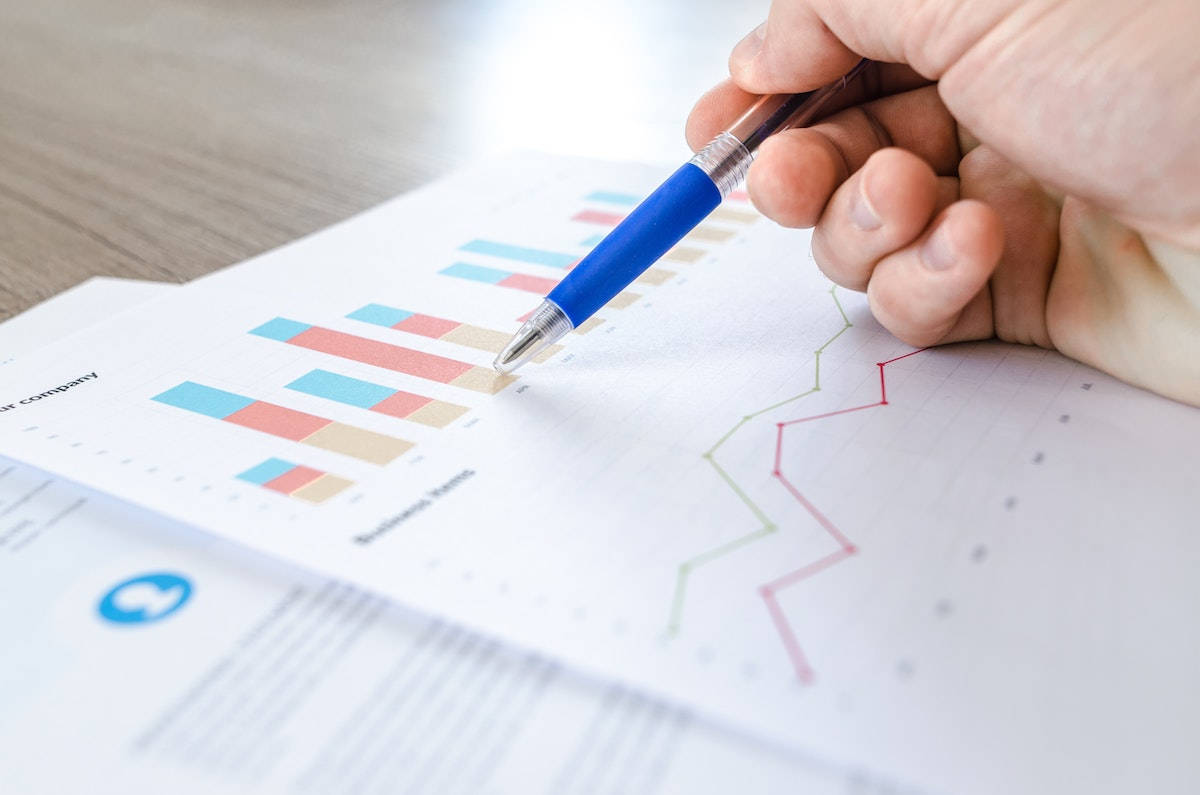Chapter 6 Data Analysis“Doing data science is building models.”

--- David Langer

Data analysis is the key task of any data science project. It can be done with many methods depending on the goal of the analysis1. The three often used methods are Descriptive analysis, Exploratory analysis, and Predictive analysis as we mention in the section ?? of chapter one. However, the dominant view of the data analysis is model building. Model building is a term used often in predictive analytics. Predictive analytics is a data analysing method that encompasses a variety of statistical techniques from data mining, predictive modelling, and machine learning together analyse current and historical facts to make predictions about future or otherwise unknown events.

Building a model is to provide a simple summary of a given dataset to reflect the data “signals” or “patterns” that are buried inside of the observed data samples. This summary sometimes called mapping. is formulated into a model. This model, once learned or constructed, can then be used to predict a future trend or particular values of a dependent variable. model building is also a frequently used term in “Machine Learning” where a machine learns a model from the observed dataset, and after verification, the model can be used for prediction on a new dataset.

1. This chapter provides basic concepts and foundations for predictive analytics if you already know them to jump to chapter 8↩︎